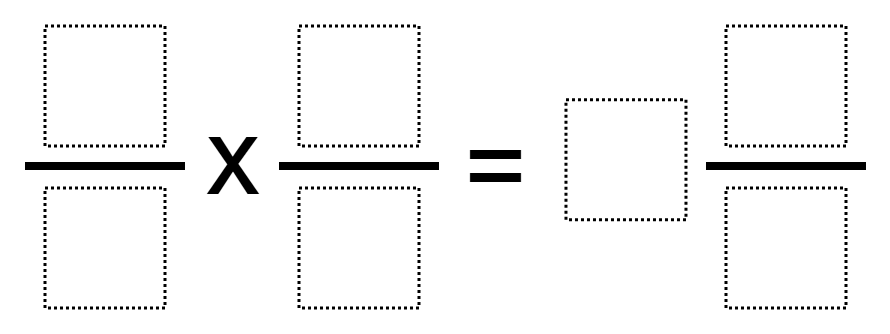Home > Grade 5 > Multiplying Fractions 4

# Multiplying Fractions 4

Directions: Using the digits 1 to 9 at most once each time, fill the boxes to make the greatest possible product.### Hint

What denominators can you use that will make a one-digit denominator?

Once answer is 9/4 * 7/3 = 5 2/8.

Source: Marc DeArmond

## Balanced Equations 2

Directions: Use the operation symbols (+, -, x, ÷) and equal sign (=) to make …

1.Amelia D'Alesandro

my problem was 8/5 x 6/4= 2 2/5. I know my numbers repeat and we have to find a way to not repeat a number but i got stuck and couldn’t think of another answer. My strategy was to use the answer given to help and then take one away from 9 making it an 8 and putting it over the 4 and taking one away from the 7 and putting it over the 3 and trying that. My strategy didn’t work. This was a really hard puzzle.

2.I had 6/2 x 3/1 = 9. I got this by finding a number that times by something would be under ten. I first got 6/2 and that got me 3. Then i got 3 from 3/1. Lastly i multiplied 3 by 3 and got 9. I agree it was a challenging puzzle.

3.•I got 9/4 x 7/3 = 5 2/8

4.Danitza Marenco

I got this 9/4×7/3=5 2/8

•good its great site
right

5.I got 9/4times 7/3=5 2/8

6.Zachary Greenberg

For my answer to that question I got 9/4 x 7/3 = 5 2/8. I got that by trying to make a very high number with the digits multiple times. At last I got one that when simplified, not all the way had no repeating digits.

7.I got 9/4 x 7/3 to equal 5 2/8. I got it by multiplying multiple ways over and over and once I got to 9/4 x 7/3, I realized 9×7= 63, 3×4=12, and 63/12= 5 3/12= 5 1/4= 5 2/8.

8.Josiah B. Fugate

9 1/3

9.i did 9/1 x 8/2= 36

10.11.I got 9/4 x 7/3 equals 1 2/8

12.I got 9/4 * 7/3 = 5 2/8 because this is the highest umber you will get while using numbers 1-9 once.

13.9/1 x 9/1 = 81/1

14.I meant : 9/1 x 8/2 = 144/2 = 72

15.I got 5 1/4

16.i got 5 2/8

17.I got 5 1/4 which changed to 5 2/8 to not repeat a number.

18.didnt mean to right this one

19.4 1/2 x 2/3= 9/2×2/3=18/3=3

20.9/4 X 7/3=63/12 =5 3/12

21.5 2/8

22.I got 3 1/6 and I had the numbers 2 &7 left over

23.24.Sophia Klawonn

I did 4/3 x 5/6 which is 7 1/2

25.Harrison Mountain

I got 9/4 x 7/3 which equals 5 2/8.

26.2/3 * 3/4 = 1 5/12
2/4 * 3/5 = 1 2/20

27.Marjorie Allred

I did 7/3 *4/1=9 2/6

28.29.4/1 * 7/3 = 9 2/6

•I had a student give me 6/4 * 7/1= 9 3/2